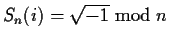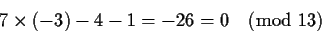Next: Square roots of integers Up: Modular mappings Previous: Arbitrary powers

## Complex numbers

Working with complex numbers reduces to being able to compute the signature of i, the imaginary unit. Sn(i) should satisfy the basic relation of Sn(i)2+1=0. This means that. If n = 4k+1 is a prime, then -1is a non-residue, and we can find its square root. If n=n1 n2 ... is composite, then -1 should be a non-residue for all ni. As a consequence n has two possible forms, n=4k+1 or n=8k+2. Once that we have chosen n so that Sn(i) has a representation, we still have two choices (plus and minus). Any choice works provided that we use it consistently. This choice is not yet a problem, but will become one once that we introduce algebraic numbers in general. This will be discussed later.

Suppose we want to test whether (x+i)(x-i)-x2-1 is identically 0. We can work with n=13, where S(i)=5 is a possible choice. Selecting S(x)=2 as before we obtainWhen we are forced to compute signatures in a field or congruence which does not allow the representation of i, we will use field extensions. This will be described for the signatures of ex and trigonometric functions.Next: Square roots of integers Up: Modular mappings Previous: Arbitrary powers
Gaston Gonnet
1999-07-04